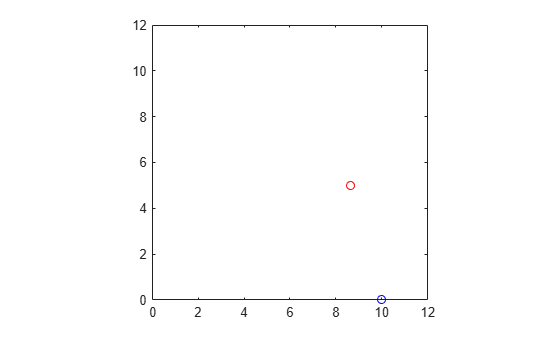Documentation

# affine2d

2-D affine geometric transformation

## Description

An `affine2d` object stores information about a 2-D affine geometric transformation and enables forward and inverse transformations.

## Creation

You can create an `affine2d` object using the following methods:

• `imregtform` — Estimates a geometric transformation that maps a moving image to a fixed image using similarity optimization

• `imregcorr` — Estimates a geometric transformation that maps a moving image to a fixed image using phase correlation

• `fitgeotrans` — Estimates a geometric transformation that maps pairs of control points between two images

• `randomAffine2d` — Creates a randomized 2-D affine transformation

• The `affine2d` function described here

### Syntax

``tform = affine2d``
``tform = affine2d(A)``

### Description

````tform = affine2d` creates an `affine2d` object with default property settings that correspond to the identity transformation.```

example

````tform = affine2d(A)` sets the property `T` with a valid affine transformation defined by nonsingular matrix `A`.```

## Properties

expand all

Forward 2-D affine transformation, specified as a nonsingular 3-by-3 numeric matrix.

The matrix `T` uses the convention:

`[x y 1] = [u v 1] * T`

where `T` has the form:

``` [a b 0; c d 0; e f 1]; ```

The default of `T` is the identity transformation.

Data Types: `double` | `single`

Dimensionality of the geometric transformation for both input and output points, specified as the value `2`.

## Object Functions

 `invert` Invert geometric transformation `isRigid` Determine if transformation is rigid transformation `isSimilarity` Determine if transformation is similarity transformation `isTranslation` Determine if transformation is pure translation `outputLimits` Find output spatial limits given input spatial limits `transformPointsForward` Apply forward geometric transformation `transformPointsInverse` Apply inverse geometric transformation

## Examples

collapse all

Create an `affine2d` object that defines a 30 degree rotation in the counterclockwise direction around the origin.

```theta = 30; tform = affine2d([cosd(theta) sind(theta) 0;... -sind(theta) cosd(theta) 0; 0 0 1])```
```tform = affine2d with properties: Dimensionality: 2 T: [3x3 double] ```

Apply the forward geometric transformation to a point (10,0).

`[x,y] = transformPointsForward(tform,10,0)`
```x = 8.6603 ```
```y = 5 ```

Validate the transformation by plotting the original point (in blue) and the transformed point (in red).

```figure plot(10,0,'bo',x,y,'ro') axis([0 12 0 12]); axis square;```Read an image into the workspace.

`A = imread('pout.tif');`

Create an `affine2d` object that defines an affine geometric transformation. This example combines vertical shear and horizontal stretch.

`tform = affine2d([2 0.33 0; 0 1 0; 0 0 1])`
```tform = affine2d with properties: Dimensionality: 2 T: [3x3 double] ```

Apply the geometric transformation to the image using `imwarp`.

`B = imwarp(A,tform);`

Display the resulting image.

```figure imshow(B); axis on equal;```